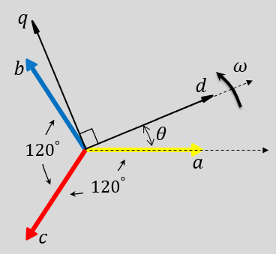# Inverse Park Transform

Implement dq0 to abc transform

•Libraries:
Simscape / Electrical / Control / Mathematical Transforms

## Description

The Inverse Park Transform block converts the time-domain direct, quadrature, and zero components in a rotating reference frame to the components of a three-phase system in an abc reference frame. The block can preserve the active and reactive powers with the powers of the system in the rotating reference frame by implementing an invariant version of the Park transform. For a balanced system, the zero component is equal to zero.

You can configure the block to align the a-axis of the three-phase system to either the d- or q-axis of the rotating reference frame at time, t = 0. The figures show the direction of the magnetic axes of the stator windings in an abc reference frame and a rotating d-q reference frame where:

• The a-axis and the q-axis are initially aligned.• The a-axis and the d-axis are initially aligned.In both cases, the angle θ = ωt, where

• θ is the angle between the a and q axes for the q-axis alignment or the angle between the a and d axes for the d-axis alignment.

• ω is the rotational speed of the d-q reference frame.

• t is the time, in s, from the initial alignment.

The figures show the time-response of the individual components of equivalent balanced dq0 and abc for an:

• Alignment of the a-phase vector to the q-axis• Alignment of the a-phase vector to the d-axis### Defining Equations

The Inverse Park Transform block implements the transform for an a-phase to q-axis alignment as

`$\left[\begin{array}{c}a\\ b\\ c\end{array}\right]=\left[\begin{array}{ccc}\text{sin}\left(\theta \right)& \text{cos}\left(\theta \right)& 1\\ \text{sin}\left(\theta -\frac{2\pi }{3}\right)& \text{cos}\left(\theta -\frac{2\pi }{3}\right)& 1\\ \text{sin}\left(\theta +\frac{2\pi }{3}\right)& \text{cos}\left(\theta +\frac{2\pi }{3}\right)& 1\end{array}\right]\left[\begin{array}{c}d\\ q\\ 0\end{array}\right],$`

where:

• d and q are the components of the two-axis system in the rotating reference frame.

• a, b, and c are the components of the three-phase system in the abc reference frame.

• 0 is the zero component of the two-axis system in the stationary reference frame.

For a power invariant a-phase to q-axis alignment, the block implements the transform using this equation:

`$\left[\begin{array}{c}a\\ b\\ c\end{array}\right]=\sqrt{\frac{2}{3}}\left[\begin{array}{ccc}\text{sin}\left(\theta \right)& \text{cos}\left(\theta \right)& \sqrt{\frac{1}{2}}\\ \text{sin}\left(\theta -\frac{2\pi }{3}\right)& \text{cos}\left(\theta -\frac{2\pi }{3}\right)& \sqrt{\frac{1}{2}}\\ \text{sin}\left(\theta +\frac{2\pi }{3}\right)& \text{cos}\left(\theta +\frac{2\pi }{3}\right)& \sqrt{\frac{1}{2}}\end{array}\right]\left[\begin{array}{c}d\\ q\\ 0\end{array}\right].$`

For an a-phase to d-axis alignment, the block implements the transform using this equation:

`$\left[\begin{array}{c}a\\ b\\ c\end{array}\right]=\left[\begin{array}{ccc}\text{cos}\left(\theta \right)& -\text{sin}\left(\theta \right)& 1\\ \text{cos}\left(\theta -\frac{2\pi }{3}\right)& -\text{sin}\left(\theta -\frac{2\pi }{3}\right)& 1\\ \text{cos}\left(\theta +\frac{2\pi }{3}\right)& -\text{sin}\left(\theta +\frac{2\pi }{3}\right)& 1\end{array}\right]\left[\begin{array}{c}d\\ q\\ 0\end{array}\right].$`

The block implements a power invariant a-phase to d-axis alignment as

`$\left[\begin{array}{c}a\\ b\\ c\end{array}\right]=\sqrt{\frac{2}{3}}\left[\begin{array}{ccc}\text{cos}\left(\theta \right)& -\text{sin}\left(\theta \right)& \sqrt{\frac{1}{2}}\\ \text{cos}\left(\theta -\frac{2\pi }{3}\right)& -\text{sin}\left(\theta -\frac{2\pi }{3}\right)& \sqrt{\frac{1}{2}}\\ \text{cos}\left(\theta +\frac{2\pi }{3}\right)& -\text{sin}\left(\theta +\frac{2\pi }{3}\right)& \sqrt{\frac{1}{2}}\end{array}\right]\left[\begin{array}{c}d\\ q\\ 0\end{array}\right].$`

## Ports

### Input

expand all

Direct-axis and quadrature-axis components and the zero component of the system in the rotating reference frame.

Data Types: `single` | `double`

Angular position of the rotating reference frame. The value of this parameter is equal to the polar distance from the vector of the a-phase in the abc reference frame to the initially aligned axis of the dq0 reference frame.

Data Types: `single` | `double`

### Output

expand all

Components of the three-phase system in the abc reference frame.

Data Types: `single` | `double`

## Parameters

expand all

Option to preserve the active and reactive power of the abc reference frame.

Align the a-phase vector of the abc reference frame to the d- or q-axis of the rotating reference frame.

 Krause, P., O. Wasynczuk, S. D. Sudhoff, and S. Pekarek. Analysis of Electric Machinery and Drive Systems. Piscatawy, NJ: Wiley-IEEE Press, 2013.